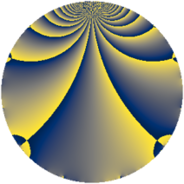# Properties

 Label 3630.2.dLevel $3630$ Weight $2$ Character orbit 3630.d Rep. character $\chi_{3630}(1451,\cdot)$ Character field $\Q$ Dimension $144$ Sturm bound $1584$

# Related objects

## Defining parameters

 Level: $$N$$ $$=$$ $$3630 = 2 \cdot 3 \cdot 5 \cdot 11^{2}$$ Weight: $$k$$ $$=$$ $$2$$ Character orbit: $$[\chi]$$ $$=$$ 3630.d (of order $$2$$ and degree $$1$$) Character conductor: $$\operatorname{cond}(\chi)$$ $$=$$ $$33$$ Character field: $$\Q$$ Sturm bound: $$1584$$

## Dimensions

The following table gives the dimensions of various subspaces of $$M_{2}(3630, [\chi])$$.

Total New Old
Modular forms 840 144 696
Cusp forms 744 144 600
Eisenstein series 96 0 96

## Trace form

 $$144q - 4q^{3} + 144q^{4} - 4q^{9} + O(q^{10})$$ $$144q - 4q^{3} + 144q^{4} - 4q^{9} - 4q^{12} - 8q^{15} + 144q^{16} - 144q^{25} + 44q^{27} + 8q^{31} - 4q^{36} + 32q^{37} + 16q^{42} - 4q^{48} - 144q^{49} - 8q^{60} + 144q^{64} + 24q^{67} - 16q^{69} + 4q^{75} - 20q^{81} + 24q^{82} - 48q^{91} - 32q^{93} + 168q^{97} + O(q^{100})$$

## Decomposition of $$S_{2}^{\mathrm{new}}(3630, [\chi])$$ into newform subspaces

The newforms in this space have not yet been added to the LMFDB.

## Decomposition of $$S_{2}^{\mathrm{old}}(3630, [\chi])$$ into lower level spaces

$$S_{2}^{\mathrm{old}}(3630, [\chi]) \cong$$ $$S_{2}^{\mathrm{new}}(33, [\chi])$$$$^{\oplus 8}$$$$\oplus$$$$S_{2}^{\mathrm{new}}(66, [\chi])$$$$^{\oplus 4}$$$$\oplus$$$$S_{2}^{\mathrm{new}}(165, [\chi])$$$$^{\oplus 4}$$$$\oplus$$$$S_{2}^{\mathrm{new}}(330, [\chi])$$$$^{\oplus 2}$$$$\oplus$$$$S_{2}^{\mathrm{new}}(363, [\chi])$$$$^{\oplus 4}$$$$\oplus$$$$S_{2}^{\mathrm{new}}(726, [\chi])$$$$^{\oplus 2}$$$$\oplus$$$$S_{2}^{\mathrm{new}}(1815, [\chi])$$$$^{\oplus 2}$$